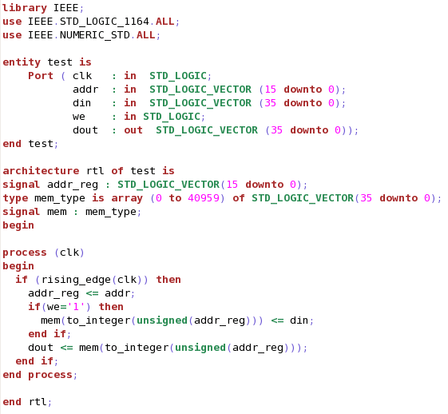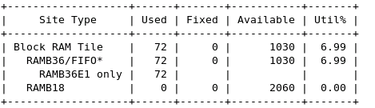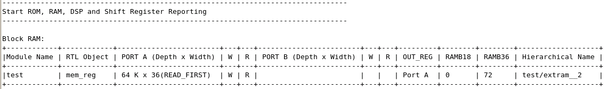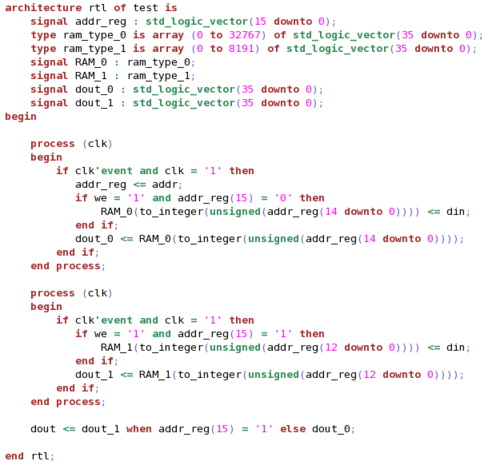# Optimizing RAMB Utilization when Memory Depth is not a Power of 2 - 2021.2 English

## Vivado Design Suite User Guide: Design Analysis and Closure Techniques (UG906)

Document ID
UG906
Release Date
2021-10-27
Version
2021.2 English

The following test case can be used to observe the log file generated by the synthesis tool and see if there is any improvement that can be done to the RTL to guide the tool in a better way. The following code snippet shows a 40K-deep 36-bit wide memory description in VHDL. The address bus requires 16 bits.

Figure 1. 40K x 36 bits Memory RTL ExampleUsing the `report_utilization` command post-synthesis, you can see that 72 block RAMs are generated by the synthesis tool, as shown in the following figure.

Figure 2. Number of Block RAMs Generated by Synthesis in the Utilization ReportIf you calculate the number of block RAMs that are supposed to be inferred for the 40K x 36 configuration, you would end up with fewer block RAMs than the synthesis tool generated.

The following shows the manual calculation for this memory configuration:

• 40K x 36 can be broken in two memories: (32K x 36) and (8K x 36)
• An address decoder based on the MSB address bits is required to enable one or the other memory for read and write operations, and select the proper output data.
• The 32K x 36 memory can be implemented with 32 RAMBs: 4 * 8 * (4K x 9)
• The 8K x 36 memory can be implemented with 8 RAMBs: 8 * (1K x 36)
• In total, 40 RAMBs are required to optimally implement the 40K x 36 memory.

To verify that the optimal number of RAMBs have been inferred, the synthesis log file includes a section that details how each memory is configured and mapped to FPGA primitives. As shown in the following figure, memory depth is treated as 64K, which gives a clue that non-power of 2 depths are not handled in an optimal way.

Figure 3. RAM Configuration and Mapping Section in the Synthesis LogThe synthesis tool has used 64K x 1 (2 block RAMs with cascade feature), 36 such structures because of 36-bit data. So in total, you have 36 x 2 = 72 block RAMs. The following figure shows the code snippet that forces synthesis to infer the optimal number of RAMBs.

Figure 4. Optimized 40 K x 36 bits Memory RTL Example